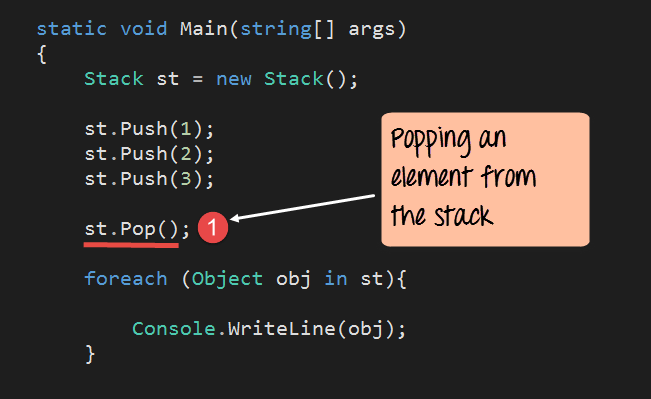## What is Stack in C#?

The stack is a special case collection which represents a last in first out (LIFO) concept. To first understand LIFO, let's take an example. Imagine a stack of books with each book kept on top of each other.

The concept of last in first out in the case of books means that only the top most book can be removed from the stack of books. It is not possible to remove a book from between, because then that would disturb the setting of the stack.

Hence in C#, the stack also works in the same way. Elements are added to the stack, one on the top of each other. The process of adding an element to the stack is called a push operation. To remove an element from a stack, you can also remove the top most element of the stack. This operation is known as pop.

Let's look at the operations available for the Stack collection in more detail.

### Declaration of the stack

A stack is created with the help of the Stack Data type. The keyword "new" is used to create an object of a Stack. The object is then assigned to the variable st.

`Stack st = new Stack()`

### Adding elements to the stack

The push method is used to add an element onto the stack. The general syntax of the statement is given below.

`Stack.push(element)`

### Removing elements from the stack

The pop method is used to remove an element from the stack. The pop operation will return the topmost element of the stack. The general syntax of the statement is given below

` Stack.pop()`

### Count

This property is used to get the number of items in the Stack. Below is the general syntax of this statement.

`Stack.Count`

### Contains

This method is used to see if an element is present in the Stack. Below is the general syntax of this statement. The statement will return true if the element exists, else it will return the value false.

`Stack.Contains(element)`

Now let's see this working at a code level. All of the below-mentioned code will be written to our Console application. The code will be written to our Program.cs file.

In the below program, we will write the code to see how we can use the above-mentioned methods.

### Example 1

In this example, we will see

• How a stack gets created.
• How to display the elements of the stack, and use the Count and Contain methods.
```using System;
using System.Collections;
using System.Collections.Generic;
using System.Linq;
using System.Text;

namespace DemoApplication
{
class Program
{
static void Main(string[] args)
{
Stack st = new Stack();
st.Push(1);
st.Push(2);
st.Push(3);

foreach (Object obj in st)
{
Console.WriteLine(obj);
}
Console.WriteLine(); Console.WriteLine();
Console.WriteLine("The number of elements in the stack " +st.Count);
Console.WriteLine("Does the stack contain the elements 3 "+st.Contains(3));
}
}
}```

Code Explanation:-

1. The first step is used to declare the Stack. Here we are declaring "st" as a variable to hold the elements of our stack.
2. Next, we add 3 elements to our stack. Each element is added via the Push method.
3. Now since the stack elements cannot be accessed via the index position like the array list, we need to use a different approach to display the elements of the stack. The Object (obj) is a temporary variable, which is declared for holding each element of the stack. We then use the foreach statement to go through each element of the stack. For each stack element, the value is assigned to the obj variable. We then use the Console.Writeline command to display the value to the console.
4. We are using the Count property (st.count) to get the number of items in the stack. This property will return a number. We then display this value to the console.
5. We then use the Contains method to see if the value of 3 is present in our stack. This will return either a true or false value. We then display this return value to the console.

If the above code is entered properly and the program is run the following output will be displayed.

Output:

From the output, we can see that the elements of the stack are displayed. Also, the value of True is displayed to say that the value of 3 is defined on the stack.

Note: You have noticed that the last element pushed onto the stack is displayed first. This is the topmost element of the stack. The count of stack elements is also shown in the output.

### Example 2

Now let's look at the "remove" functionality. We will see the code required to remove the topmost element from the stack.```using System;
using System.Collections;
using System.Collections.Generic;
using System.Linq;
using System.Text;

namespace DemoApplication
{
class Program
{
static void Main(string[] args)
{
Stack st = new Stack();
st.Push(1);
st.Push(2);
st.Push(3);

st.Pop();

foreach (Object obj in st)
{
Console.WriteLine(obj);
}
}
}
}```

Code Explanation:-

1. Here we just issue the pop method which is used to remove an element from the stack.

If the above code is entered properly and the program is run, the following output will be displayed.

Output:

We can see that the element 3 was removed from the stack.

Summary

• A Stack is based on the last in first out concept. The operation of adding an element to the stack is called the push operation. The operation of removing an element to the stack is called the pop operation.

YOU MIGHT LIKE: